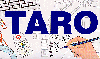The Traffic Accident Reconstruction Origin -Approach Angles Problem-[Home] [ARnews] [Contents] [Classified] [Advertisers] [Approach Angles] [E-mail Directory] [Feedback] [Organizations] [Reference Library]

The Falling Pedestrian (more correctly The Toppling Pedestrian)

by Dr. Eddie Baart

This problem was posted in TARO's Approach Angles. The question resolves to the following:

Could the pedestrian have "fallen" to a position where his waist was 10 feet from the curb?

Facts

The problem states that the pedestrian fell off the curb into the roadway. The height of this curb is unspecified. This solution will include this height as a general variable h. This general approach will also allow the solution to cover similar situations where the pedestrian could have fallen from a great height.

The top of the ledge is a vertical distance of h feet from the ground. The pedestrian was struck 10 feet horizontally from the edge of the curb. His CM is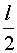off the ground where l is his overall height.

Questions

What would the pedestrian's horizontal velocity be if you assumed that his body were rigid from the feet to the CM and he tipped out away from the curb while standing on it? What would be the probable horizontal distance traveled from the edge to the point of impact?"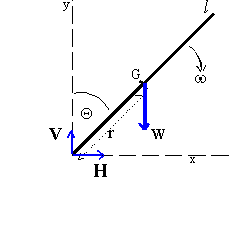The Solution

Suppose the pedestrian is of height l (l/2 is distance from center of gravity to his feet). We describe the motion of the center of gravity (G) in terms of the coordinates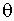the angle of lean and r as shown in the diagram. The x coordinate of G is l/2 cosso its x component of velocity (by differentiation) is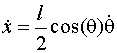Equation.(a)

and its x component of acceleration is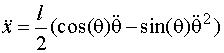Equation(1)

The two terms in the x acceleration can be recognized as the horizontal components of the tangential acceleration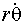and the radial (centripetal) acceleration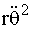There is a radial acceleration as in uniform circular motion because, although r is not changing in magnitude, its direction is changing.

The feet of the pedestrian will lose contact with the ledge when the horizontal component of the force exerted on them by the ledge (H in the diagram) becomes zero i.e. when the x component of the acceleration shown above becomes zero.

In order to find the angle at which this occurs we need to find expressions for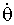and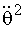. To do this we use Newton's Second Law (for rotation) and Conservation of Energy.

Newton's Second Law

For rotational motion this law states that the moment of inertia times the angular acceleration equals the torque (by analogy with F = ma for linear motion). The moment of inertia of the pedestrian for an axis through his feet is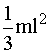So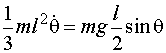and hence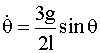Equation(2)

Conservation of EnergyThe rotational kinetic energy when the pedestrian is at an anglemust equal the gravitational potential energy it has lost as his center of mass (CM) descending from its previous height of l/2 above his feet to the new height l/2 cos. Thus,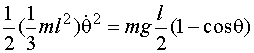and so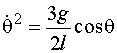Equation(3)

The Angle of Launch

We now use the condition for the horizontal force to be zero i.e. that the x acceleration of equation (1) is zero.

If we substitute equations (2) and (3) into equation (1) and set x=0 we get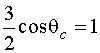and hence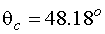It is noteworthy to observe that this result is independent of l so it applies to the pedestrian regardless of his height, or a pencil toppling off a table.

The components of launch velocity

We can get the horizontal component of his velocity at the instant the pedestrian leaves his feet by substituting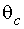into the equation (3) to findand then using equation a.

This gives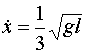Equation(4)

We can get a similar expression for the vertical speed when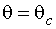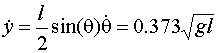Equation(5)

The Problem at Hand

We can apply equations (4) and (5) to the problem at hand. If the victim was 70 inches tall (1.778m) then l = 2 x 0.889 m (2 x 35 inches).

The height of the CM at launch is l/2 cos 48.18 above his feet = 0.593 m and vertical velocity is 1.557 m/s. So he falls 0.593m + h , where h is the curb height. If this was a normal curb of .152 m (6 inches) the victim falls 0.593 + .152 = .745 m (2.45 ft). This fall takes .39 seconds.

Since the horizontal launch velocity is 1.391 m/s (4.56 ft/s) he travels a horizontal distance of .54m (1.8 ft).

If we compare this distance to the given distance of 10 feet (3.05m), then it is obvious that this was not a simple topple with the assumptions I have used. Whether foul play occurred, I leave to your judgement.

Eddie Baart is Professor (soon to retire) of Physics at Rhodes University, Grahamstown, South Africa. He has a Ph.D. in Nuclear Physics but has done most of his research in Radio Astronomy. He does accident reconstruction as a sideline to his teaching of physics. It was by accident that he became involved in this field about 28 years ago. Since then he has reconstructed more than 300 accidents and given evidence in court more than 60 times.

He can be contacted at pheb@giraffe.ru.ac.za

[Home Site] [ARnews] [Contents] [Classified] [Advertisers] [Approach Angles] [E-mail Phone Book] [Feedback] [Organizations] [Reference Library]

v